Next: Tcl Boundary Forces Up: User Defined Forces Previous: Interactive Molecular Dynamics (IMD)   Contents   Index

## Tcl Forces and Analysis

NAMD provides a limited Tcl scripting interface designed for applying forces and performing on-the-fly analysis. This interface is efficient if only a few coordinates, either of individual atoms or centers of mass of groups of atoms, are needed. In addition, information must be requested one timestep in advance. To apply forces individually to a potentially large number of atoms, use tclBC instead as described in Sec. 9.11. The following configuration parameters are used to enable the Tcl interface:

• tclForces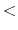is Tcl interface active?Acceptable Values: on or off
Default Value: off
Description: Specifies whether or not Tcl interface is active. If it is set to off, then no Tcl code is executed. If it is set to on, then Tcl code specified in tclForcesScript parameters is executed.

• tclForcesScriptinput for Tcl interfaceAcceptable Values: file or {script}
Description: Must contain either the name of a Tcl script file or the script itself between { and } (may include multiple lines). This parameter may occur multiple times and scripts will be executed in order of appearance. The script(s) should perform any required initialization on the Tcl interpreter, including requesting data needed during the first timestep, and define a procedure calcforces { } to be called every timestep.

At this point only low-level commands are defined. In the future this list will be expanded. Current commands are:

• print <anything>
This command should be used instead of puts to display output. For example, print Hello World''.

• atomid <segname> <resid> <atomname>
Determines atomid of an atom from its segment, residue, and name. For example, atomid br 2 N''.

Request coordinates of this atom for next force evaluation, and the calculated total force on this atom for current force evaluation. Request remains in effect until clearconfig is called. For example, addatom 4'' or addatom [atomid br 2 N]''.

Request center of mass coordinates of this group for next force evaluation. Returns a group ID which is of the form gN where N is a small integer. This group ID may then be used to find coordinates and apply forces just like a regular atom ID. Aggregate forces may then be applied to the group as whole. Request remains in effect until clearconfig is called. For example, set groupid [addgroup { 14 10 12 }]''.

• clearconfig
Clears the current list of requested atoms. After clearconfig, calls to addatom and addgroup can be used to build a new configuration.

• getstep
Returns the current step number.

Loads requested atom and group coordinates (in Å) into a local array. loadcoords should only be called from within the calcforces procedure. For example, loadcoords p'' and print $p(4)''. • loadforces <varname> Loads the forces applied in the previous timestep (in kcal molÅ) into a local array. loadforces should only be called from within the calcforces procedure. For example, loadforces f'' and print$f(4)''.

• enabletotalforces/disabletotalforces
Enables/disables the loadtotalforces'' command, described below, which is disabled by default to avoid unneeded work and communication.

Loads the total forces on each requested atom and group in the previous time step (in kcal molÅ) into a local array. The total force also includes external forces. Note that the loadforces'' command returns external forces applied by the user. Therefore, one can subtract the external force on an atom from the total force on this atom to get the pure force arising from the simulation system. Note that enabletotalforces'' must be called first.

Loads requested atom and group masses (in amu) into a local array. loadmasses should only be called from within the calcforces procedure. For example, loadcoords m'' and print $m(4)''. • addforce <atomid|groupid> <force vector> Applies force (in kcal molÅ) to atom or group. addforce should only be called from within the calcforces procedure. For example, addforce$groupid { 1. 0. 2. }''.

This command adds the specified energy to the MISC column (and hence the total energy) in the energy output. For normal runs, the command does not affect the simulation trajectory at all, and only has an artificial effect on its energy output. However, it can indeed affect minimizations.

With the commands above and the functionality of the Tcl language, one should be able to perform any on-the-fly analysis and manipulation. To make it easier to perform certain tasks, some Tcl routines are provided below.

Several vector routines (vecadd, vecsub, vecscale) from the VMD Tcl interface are defined. Please refer to VMD manual for their usage.

The following routines take atom coordinates as input, and return some geometry parameters (bond, angle, dihedral).

• getbond <coor1> <coor2>
Returns the length of the bond between the two atoms. Actually the return value is simply the distance between the two coordinates. coor1'' and coor2'' are coordinates of the atoms.

• getangle <coor1> <coor2> <coor3>
Returns the angle (from 0 to 180) defined by the three atoms. coor1'', coor2'' and coor3'' are coordinates of the atoms.

• getdihedral <coor1> <coor2> <coor3> <coor4>
Returns the dihedral (from -180 to 180) defined by the four atoms. coor1'', coor2'', coor3'' and coor4'' are coordinates of the atoms.

The following routines calculate the derivatives (gradients) of some geometry parameters (angle, dihedral).

An angle defined by three atoms is a function of their coordinates: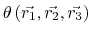(in radian). This command takes the coordinates of the three atoms as input, and returns a list of {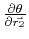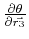}. Each element of the list is a 3-D vector in the form of a Tcl list.

• dihedralgrad <coor1> <coor2> <coor3> <coor4>
A dihedral defined by four atoms is a function of their coordinates: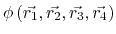(in radian). This command takes the coordinates of the four atoms as input, and returns a list of {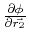}. Each element of the list is a 3-D vector in the form of a Tcl list.

As an example, here's a script which applies a harmonic constraint (reference position being 0) to a dihedral. Note that the addenergy'' line is not really necessary - it simply adds the calculated constraining energy to the MISC column, which is displayed in the energy output.

tclForcesScript {
# The IDs of the four atoms defining the dihedral
set aid1 112
set aid2 123
set aid3 117
set aid4 115

# The "spring constant" for the harmonic constraint
set k 3.0

addatom $aid1 addatom$aid2
addatom $aid3 addatom$aid4

set PI 3.1416

proc calcforces {} {

global aid1 aid2 aid3 aid4 k PI

# Calculate the current dihedral
set phi [getdihedral $p($aid1) $p($aid2) $p($aid3) $p($aid4)]
set phi [expr $phi*$PI/180]

# (optional) Add this constraining energy to "MISC" in the energy output
addenergy [expr $k*$phi*$phi/2.0] # Calculate the "force" along the dihedral according to the harmonic constraint set force [expr -$k*$phi] # Calculate the gradients foreach {g1 g2 g3 g4} [dihedralgrad$p($aid1)$p($aid2)$p($aid3)$p($aid4)] {} # The force to be applied on each atom is proportional to its # corresponding gradient addforce$aid1 [vecscale $g1$force]
addforce $aid2 [vecscale$g2 $force] addforce$aid3 [vecscale $g3$force]
addforce $aid4 [vecscale$g4 \$force]
}
}Next: Tcl Boundary Forces Up: User Defined Forces Previous: Interactive Molecular Dynamics (IMD)   Contents   Index
http://www.ks.uiuc.edu/Research/namd/